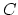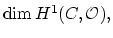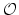# Arithmetic genus

The arithmetic genus of an irreducible, projective curveiswhereis the structure sheaf of holomorphic functions on. For a smooth curve, this is the same as the geometric genus; however, unlike the geometric genus, the arithmetic genus has the nice feature that it remains constant in families of curves with possibly singular fibers. Intuitively this means that the arithmetic genus of a nodal curve is the geometric genus of the curve obtained by smoothing out the nodes.

Jeffrey Herschel Giansiracusa 2005-06-27